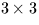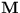Next: SLA_NUT - Nutation Matrix
Up: SUBPROGRAM SPECIFICATIONS
Previous: SLA_MXM - Multiply Matrices

## SLA_MXV - Apply 3D Rotation

ACTION:
Multiply a 3-vector by a rotation matrix (single precision).
CALL:
CALL sla_MXV (RM, VA, VB)

GIVEN:

 R(3,3) RM rotation matrix VA R(3) vector to be rotated

RETURNED:

 R(3) VB result vector

NOTES:
1.
This routine performs the operation:
b = Ma
where a and b are the 3-vectors VA and VB respectively, and M is thematrix RM.
2.
The main function of this routine is apply a rotation; under these circumstances,is a proper real orthogonal matrix.
3.
To comply with the ANSI Fortran 77 standard, VA and VB must not be the same array. The routine is, in fact, coded so as to work properly on the VAX and many other systems even if this rule is violated, something that is not, however, recommended.Next: SLA_NUT - Nutation Matrix
Up: SUBPROGRAM SPECIFICATIONS
Previous: SLA_MXM - Multiply Matrices

SLALIB --- Positional Astronomy Library